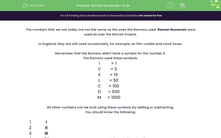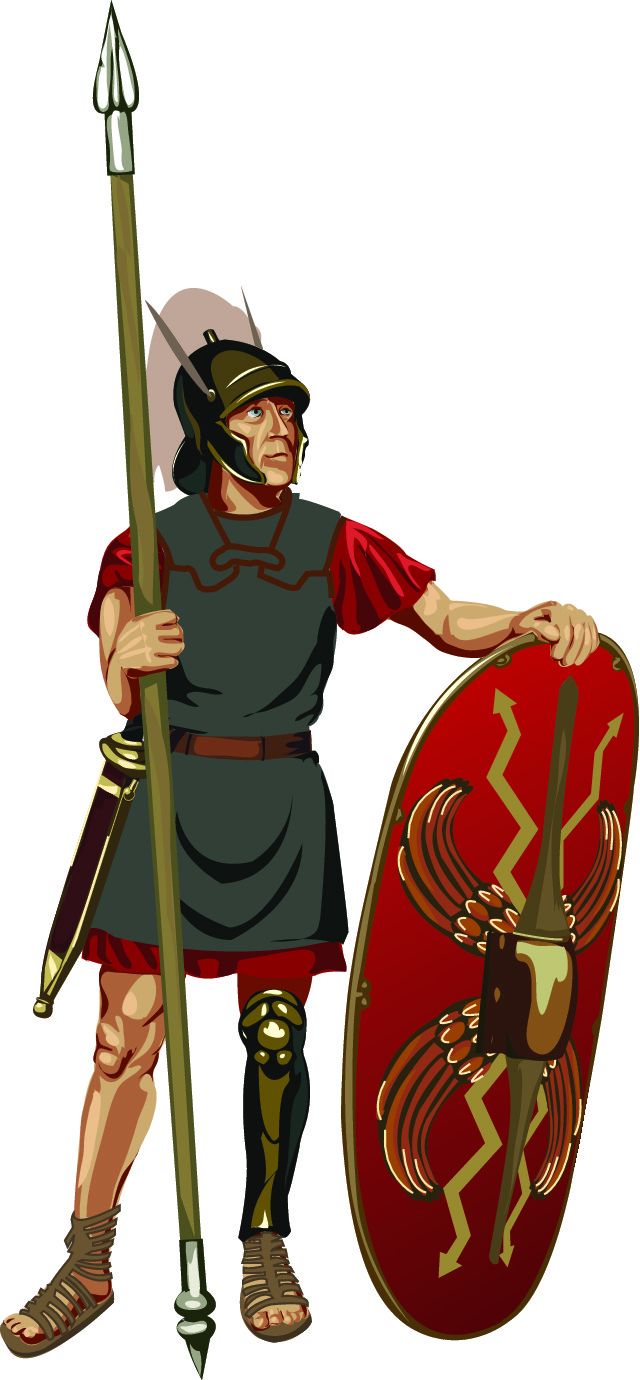# Convert to Roman Numerals

In this worksheet, students will convert normal numbers to Roman numerals up to 1000s.Key stage:  KS 2

Curriculum topic:   Number: Number and Place Value

Curriculum subtopic:   Read Roman Numerals to M (1000)

Difficulty level:#### Worksheet Overview

The numbers that we use today are not the same as the ones the Romans used. Roman numerals were used all over the Roman Empire.In England, they are still used occasionally, for example, on film credits and clock faces.

Remember that the Romans didn't have a symbol for the number 0.

The Romans used these symbols:

 I = 1 V = 5 X = 10 L = 50 C = 100 D = 500 M = 1000

All other numbers can be built using these symbols by adding or subtracting.

A letter placed after another letter means that you add the second letter to the first letter.

A letter placed before another letter means that you subtract the first letter from the second.

You should know the following:

 1 = I 2 = II 3 = III 4 = IV 5 = V 6 = VI 7 = VII 8 = VIII 9 = IX 10 = X 11 = XI 12 = XII

We build bigger numbers by adding 10s or Xs in Roman numerals.

 XX = X + X = 20 XXI = XX + I = 20 + 1 = 21 XXX = X + X + X = 30 XXXVII = XXX + VII = 30 + 7 = 37 XL =  50 - 10 = 40 XLVIII = XL + VIII = 40 + 8 = 48 XLIX = XL + IX = 40 + 9 = 49 LIV = L + IV = 50 + 4 = 54 LX =  50 + 10 = 60 LXVII = LX + VII = 60 + 7 = 67 LXX = L + XX = 50 + 20 = 70 LXXX = L + XXX = 50 + 30 = 80 LXXIV = L + XXXIV = 50 + 34 = 84 XC = 100 - 10 = 90 XCVI = XC + VI = 90 + 6 = 96

We build even bigger numbers by adding 100s, 500s or 1000s or Cs, Ds and Ms in Roman numerals.

 CC = C + C = 200 CCCXI = C + C + C + X + I = 300 + 10 + 1 = 311 CD = 500 - 100 = 400 CDXLIV = CD + XL + IV = 400 + 40 + 4 = 444 D = 500 DCCC = D + C + C + C = 500 + 300 = 800 CM =  1000 - 100 = 900 M = 1000

Larger numbers are built from smaller numbers.

 MCC = M + C + C = 1000 + 200 = 1200 MCCCI = M + C + C + C + X + I = 1000 + 300 + 1 = 1311 MCD = M + CD = 1000 + 400 = 1400 MCM = M + CM = 1000 + 900 = 1900

Example

Convert 1895 and 1948 to Roman numerals.

Build the numbers using smaller numbers that you know.

Then string them together in a line.

1895 = 1000 + 800 + 90 + 5 = M + DCCC + XC + V = MDCCCXCV

1948 = 1000 + 900 + 40 + 8 = M + CM + XL + VIII = MCMXLVIII

You can check back to this page at any point during the activity by clicking on the red help button on the screen.### What is EdPlace?

We're your National Curriculum aligned online education content provider helping each child succeed in English, maths and science from year 1 to GCSE. With an EdPlace account you’ll be able to track and measure progress, helping each child achieve their best. We build confidence and attainment by personalising each child’s learning at a level that suits them.

Get started